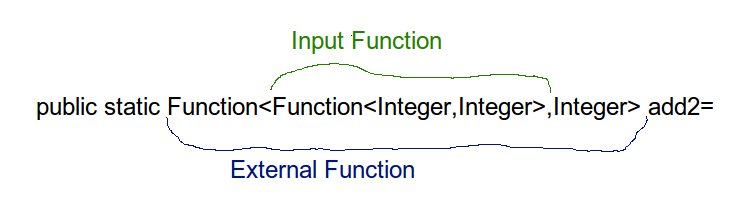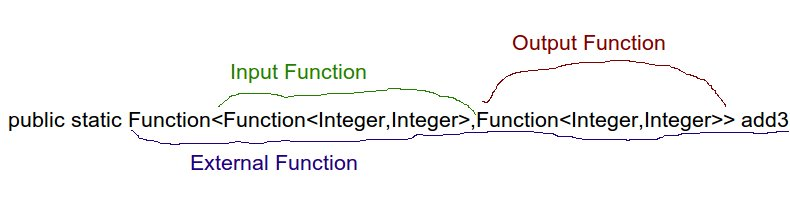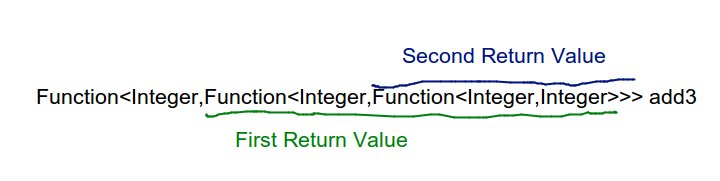# Workshop - Functional Programming Java8 - an introduction.

This is the first workshop from the Java 8 Functional Programming educational cycle. It will be mainly based on those two books

To make education more interesting and effective I decided to split material for this workshops into 3 parts:

1. Practical short example to illustrate small real life usage of this approach. This way people can see usefulness of FP programming and gain proper motivation
2. Short exercises - fast action <--> reward cycle should make learning a lot more interesting. There are two main areas which we are going to practice:
1. Functions composition
2. What is currying
3. Some theory - to gain deeper knowledge of what actually we are doing.
4. Homework! - yes you need to practice, practice, practice !!!

If you find any errors int his material please let me know so I will fix it.

#### Git repo

``````git clone https://github.com/PawelWlodarski/workshops.git
``````

And then go to the package : https://github.com/PawelWlodarski/workshops/tree/master/src/main/java/workshops/functional/fp1/

# First Practical Example

Source code of the first exercise can be found here :

https://github.com/PawelWlodarski/workshops/blob/master/src/main/java/workshops/functional/fp1/exercises/FP1PracticalExample.java

#### Educational valueIt is important to understand what potential educational value each part of this exercise tries to give to students. The code you are going to see is optimized "towards educational experience". In this example we are going to extract some data from a datastore imitating real database with missing data. And then we will use this optional data in a computation - composed of two pure functions from completely different contexts/libraries.

First Explain the Domain!Now Explain Functional Composition

For example this part :

``````
static Function<Purchase,Collection<BigDecimal>> domainFunction = null;

static Function<Collection<BigDecimal>, BigDecimal> genericMathFunction=null;
``````

Shows that in your application you can easily divide functionality between specialized functions. So we have a dedicated "domain" function which solves a problem from specific field and also we have a very general math function which can be used in many diferent contexts.

And now look at this part:

``````static private Map<Integer,Purchase> database=initDatabase();

public static Optional<Purchase> findPurchase(Integer id){
throw new UnsupportedOperationException();
}
``````

Here even without implementaiton the Type system is telling us "look I can not promise that I'm able to compute something meaningfull from your arguments - so optionally I may return some result"

And one more thing - in Purchase class :

``````class Purchase{
final Integer id;
private final List<Product> purchasedProducts;

public Purchase(final Integer id, final List<Product> purchasedProducts) {
this.id = id;
this.purchasedProducts = new ArrayList<>(purchasedProducts);
}

public List<Product> getPurchasedProducts() {
return Collections.unmodifiableList(purchasedProducts) ;
}
}
``````

We can see that id is not private. This may be considered as an antipattern from OOP point of view but here we made it final so it can be safely accessed by many concurrent threads. On the other hand the "List field" is mutable as a data structure so we took care of wraping it properly within accessor method and we made reference to it private.

It is important for students to stop mechanically repeat old Java pattern "private field+getter/setter" and go deeper into analysis if given fields represent just a value or it is actually dangerous mutable state? Should it be mutable or not? what are profits and consequences?

Now let's take a look at a potential usage :

``````public static void main(String[] args){
Function<Purchase,BigDecimal> pureDomainFunction=domainFunction.andThen(genericMathFunction);

findPurchase(1).map(pureDomainFunction).ifPresent(result-> System.out.println("sum for purchase 1 : "+result));

BigDecimal priceOfPurchase3 = findPurchase(3).map(pureDomainFunction).orElseThrow(() -> new RuntimeException("there is no purchase with id 3"));
System.out.println("somehow we have found price of purchase 3 : "+priceOfPurchase3);
}
``````

In the code displayed above we can see some examples of:

• functional composition which preserve "functional purity"
• how functional Type allow us to use pure logic in inpure context
• possible outcomes of this per&inpure interaction

We will discuss about this code during workshops but like it was mentioned before at this point it is more important to see some usefulness of functional approach than to understand all the details.

#### Look at unit tests

There are two unit tests for both domain and general functionl. They are there to help validate exercise solutions.

``````@Test
public void shouldExtractAllPricesFromPurchase(){
Purchase sut=preparePurchase();

Collection<BigDecimal> result = FP1PracticalExample.domainFunction.apply(sut);

assertThat(result,
hasItems(new BigDecimal("3000"),new BigDecimal("120"),new BigDecimal("70"),new BigDecimal("200"))
);
}

@Test
public void shouldSumCollectionOfBigDecimals(){
Collection<BigDecimal> bigDecimals=Arrays.asList(new BigDecimal("3000"),new BigDecimal("120"),new BigDecimal("70"),new BigDecimal("200"));

BigDecimal result = FP1PracticalExample.genericMathFunction.apply(bigDecimals);

assertThat(result,is(new BigDecimal("3390")));
}
``````

Exercise : implement missing functions

# Exercises

## Functions composition

https://github.com/PawelWlodarski/workshops/blob/master/src/main/java/workshops/functional/fp1/exercises/FP1FunctionsComposition.java

#### Create our own functional interface

To understand what is @FunctionalInterface in Java8

#### write custom "andThen"

``````static <A,B,C> Function<A,C> andThen(Function<A,B> f1, Function<B,C> f2)
``````

Carefully look at types. Try to use A,B,C to understand order of computation

#### write custom "compose"

This one is similar to andThen (and actually compose is the original way of composing function - that's why it is called ... composition)

``````static <A,B,C> Function<A,C> compose(Function<B,C> f1,Function<A,B> f2)
``````

``````static <A> Function<A,A> composeCollection(Collection<Function<A,A>> functions){
}
``````

Here we are going to address a practical problem of Java8 syntax. When you start writing high order functions which accept and returns functions you quickly observe that number of diamonds operator will make code less and less readable.

### Implement function with simple types

``````public static Function<Integer,Integer> add1
``````

### Implement function with Function input type and simple output type

When calling argument function just pass value 1.

``````public static Function<Function<Integer,Integer>,Integer> add2
``````### Implement Function with function as an input and function as an output

``````public static Function<Function<Integer,Integer>,Function<Integer,Integer>> add3
``````### Use Aliases to improve readability

``````public static Function<Int_to_Int,Int_to_Int> add3Aliases
``````

``````//change function so that input parameter is printed out
public static Function<Function<Integer,Integer>,Function<Integer,Integer>> decorateLogging

//Decorate function Int=>Int so that it is able to receive string parameter and parse it internally
``````

## Currying

It is very important to notice here that as an input we always have a simple value (in this example Integer) and functions values are outputs - that way we can simulate functional application with multiple arguments.

This concept should become more intuitive after some exercises.

### implement simple one argument function

``````Function<Integer,Integer> add1
``````

### implement Function which return Function

``````Function<Integer,Function<Integer,Integer>> add2
``````

### implement function which returns function which returns function

``````public static Function<Integer,Function<Integer,Function<Integer,Integer>>> add3
``````### implement function which returns function which returns function which return a function``````Function<Integer,Function<Integer,Function<Integer,Function<Integer,Integer>>>> add4
``````

### Not a function but somehow a function

Till now we were using proper mathematical functions y=f(x) which has one parameter and one possible result.

It is possible to define in Java8 a function which apply to more than one arguments. You can do it on your own

``````@FunctionalInterface
interface Function2<A,B,C> {
C apply(A a,B b);
}

``````

or just use build in Java8 BiFunction

``````public static BiFunction<Integer,Integer,Integer> biFunction=(a, b)->a+b;
``````

So why we need to use currying when we can define "a function" with more than one argument? Currying allows you to use many advanced FP techniques, it allows you to use partial applciation and even to implement with pure lambdas construct added in Java7 called "try with resources" (loan pattern). We just started so some patience is needed.

# Some theory imeprative/pure/functional

• Mutable numbers - global state changed

Observation - when we see that an operation doesn't return anything (void) then it has to change some state somewhere ("some" and "somewhere" - a dangerous situation)

``````class MutableNumber{
private int value=0;

public MutableNumber(int value) {
this.value = value;
}

public void increment(){
value=value+1;
}

public void decrement(){
value=value-1;
}

public int getValue() {
return value;
}
}

public static MutableNumber add(ObiektNumer a, ObiektNumer b) {
while (b.moreThan(0)) {
a.increment();
b.decrement();
}
return a;
}
``````
• mutable inside / mutation not observed outside
``````public static int add(int a, int b) {
while (b > 0) {
a++;
b--;
}
return a;
}
``````
• not mutable but observed effect (exception)
``````public static int div(int a, int b) {
return a / b;
}
``````
• not mutable but observed expplicit external effect (console)
`````` public static int addWithConsole(int a, int b) {
while (b > 0) {
a++;
b--;
}
System.out.println(a);
return a;
}
``````

# Referential Transparency

What is referential transparency and what we losing when RF is lost?

• RF is lost when there are observable side effects
• it may be impossible to compose operations because you are also composing side effects
• operations may be context dependant and unusable in different places
• each invocation may returns different result so you can not use Property Based Testing
• one operation can actually change behaviour of some other operation
• time dependant!
• you need to mock side effects

# Homework!!!

clone "Functional programming in Java" books repo :

https://github.com/fpinjava/fpinjava/tree/master/fpinjava-parent

Try to complete exercises from books chapter two :

https://github.com/fpinjava/fpinjava/tree/master/fpinjava-parent/fpinjava-usingfunctions-exercises/src/main/java/com/fpinjava/functions

And use test to validate results

https://github.com/fpinjava/fpinjava/tree/master/fpinjava-parent/fpinjava-usingfunctions-exercises/src/test/java/com/fpinjava/functions